Win up to 100% scholarship on Aakash BYJU'S JEE/NEET courses with ABNAT Win up to 100% scholarship on Aakash BYJU'S JEE/NEET courses with ABNAT

# Wave Optics in Physics

Wave optics, also called physical optics, deals with the study of various phenomena such as polarization, diffraction, interference and other occurrences where ray approximation of geometric optics cannot be done. Thus, the section of optics that deals with the behaviour of light and its wave characteristics is said to be wave optics.

In wave optics, the approximation is carried out by using ray optics for the estimation of the field on a surface. Further, it involves integrating ray-estimated field over a mirror, lens or aperture for the calculation of the scattered or transmitted field. We will learn about the concept of wave optics for IIT JEE in detail here.

## Wave Optics Theories

Wave optics stands as a witness to a famous standoff between two great scientific communities who dedicated their lives to understanding the nature of light. One supports the particle nature of light, and the other supports the wave nature.

Sir Isaac Newton stands as a prominent figure who supported the voice of the particle nature of light; he proposed a corpuscular theory, which states that “light consists of extremely light and tiny particles known as corpuscles which travel with very high speeds from the source of light to create a sensation of vision by reflecting on the retina of the eye”.

Also Read: Young’s Double Slit Experiment

Using this theory, Newton was able to explain reflection and refraction but failed to explain the cause of interference, diffraction and polarization. The major failure of Newton’s corpuscular theory was that it could not explain why the velocity of light was lesser in the denser medium compared to the vacuum.

## Huygens Wave Theory

No one dared to challenge Newton’s corpuscular theory until Christopher Huygens proposed his wave theory of light in the early 18th century. According to Huygens’s theory, light consists of waves that travel through a very dilute and highly elastic material medium present everywhere in space”. This medium is called ether.

As the medium is supposed to be very dilute and highly elastic, its density would be very low, and the modulus of elasticity would be very high so that the speed of the light would be very large.

Huygens’s wave theory explained phenomena like reflection, refraction, interference, and diffraction of light. But failed to explain the following:

1. Polarization, as Huygens assumed that light waves, which are longitudinal in nature, are mechanical disturbances.
2. Black body radiation, photoelectric effect and Compton effect.
3. Hypothetical medium ether was never discovered, and now we know light can propagate in a vacuum.

## Maxwell Electromagnetic Theory

According to Maxwell, light is not a mechanical wave. It is an electromagnetic wave which is transverse in nature and travels with a finite speed, given by,

$$\begin{array}{l}C=\frac{1}{\sqrt{{{\mu }_{0}}{{\varepsilon }_{0}}}}=3\times {{10}^{8}}{}^{m}/{}_{s}\end{array}$$

μo is the permeability = 4π x 10-7

εo is the permittivity = 8.854 x 10-12

## Wavefront and Wave Normal

### What Is Wavefront?

A wavefront is defined as the locus of all points of the medium which vibrate in the same phase. Depending on the shape of the source of light, wavefronts can be of three types.

#### Spherical Wave Front

When light emerges from a point source, the wavefronts are spherical in shape.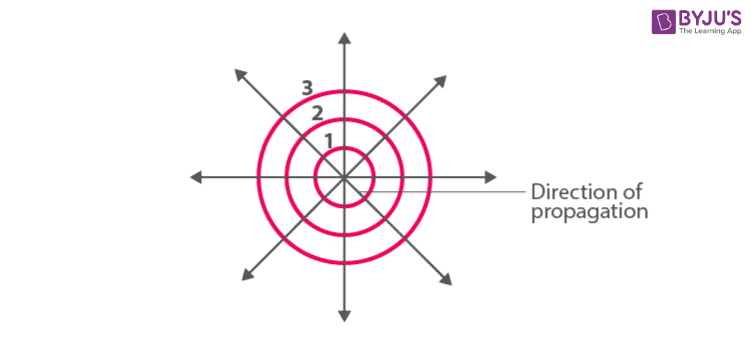In spherical wavefront,

Amplitude of light waves,

$$\begin{array}{l}A\propto \frac{1}{r}\end{array}$$

And, Intensity of light waves,

$$\begin{array}{l}I\propto \frac{1}{{{r}^{2}}}\end{array}$$

#### Cylindrical Wave Front

When the source of light is linear, the wavefronts are cylindrical in shape. All the points are equidistant from the source.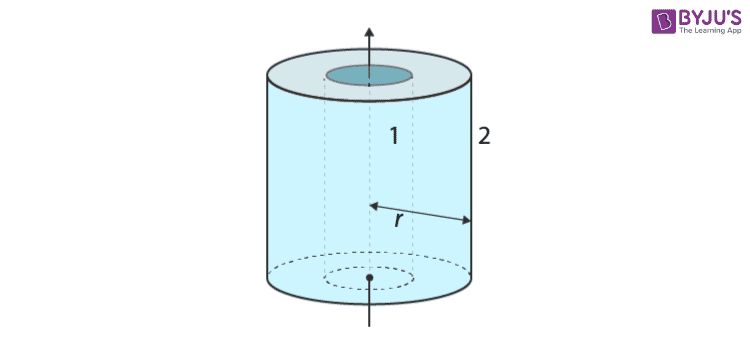In this case, the amplitude of light waves is

$$\begin{array}{l}A\propto \frac{1}{\sqrt{r}}\end{array}$$

Intensity of light waves is

$$\begin{array}{l}I\propto \frac{1}{r}\end{array}$$

#### Plane Wave Front

When the light is coming from a very far-off source, the wavefronts are planar. For a plane wavefront, the amplitude remains constant; therefore, the intensity remains constant.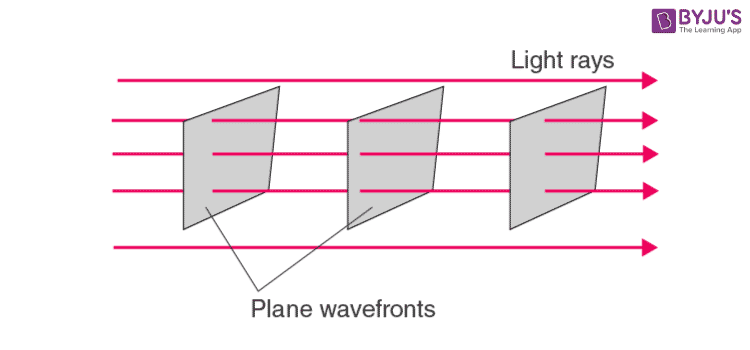### Optics Important JEE Main Questions### Wave Optics – Top 10 Most Important and Expected JEE Questions### What Is Wave Normal?

A perpendicular drawn to the surface of a wavefront at any point in the direction of propagation of light is called “wave normal”. The direction in which light travels is called a ‘ray’ of light. Thus, a wave normal is the same as a ray of light.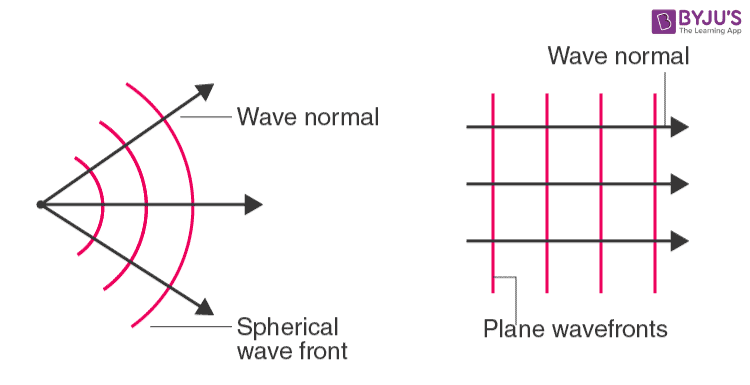### Shape of Wavefronts

A lens can be used to change the shape of wavefronts. The concept of wavefronts for reflection and refraction is explained below.

#### Wavefronts for Reflection

• If light falls on a plane mirror

If the plane wavefronts are being reflected on the plane mirror, the shape of the wavefront of the reflected light is again planar.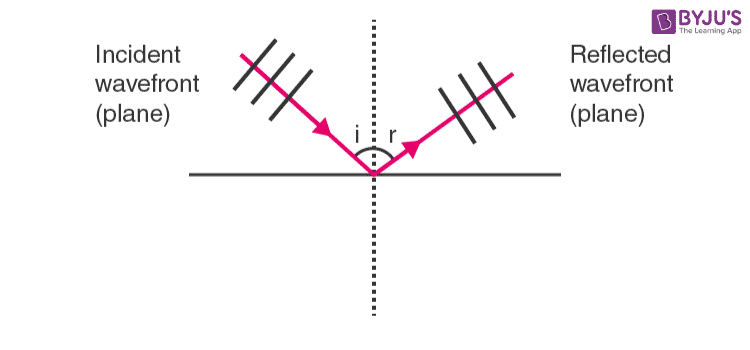• If light falls on a concave mirror

If a plane wavefront falls on a concave mirror, the shape of the reflected light is spherical.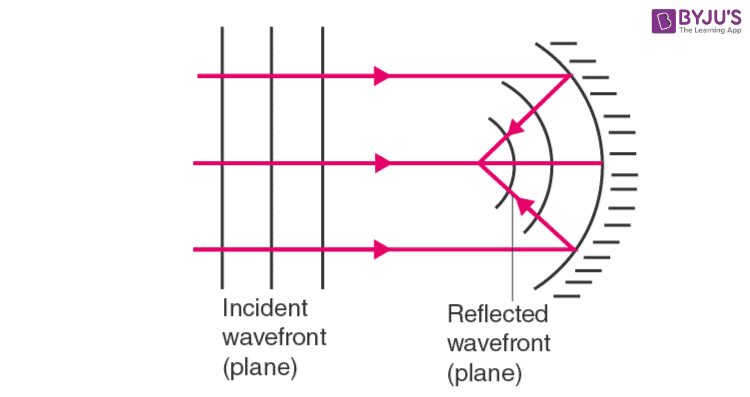• If light falls on a convex mirror

If a plane wavefront falls on a convex mirror, the shape of the reflected light is spherical.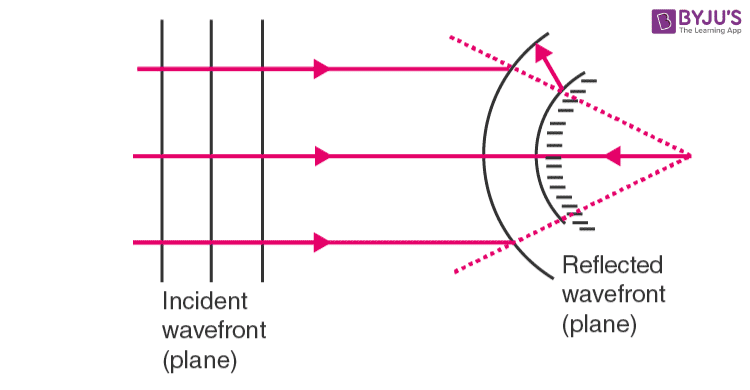#### Wavefronts for Refraction

• If light falls on plane surfaces

If a plane wavefront falls on a plane surface, the refracted ray will also have a plane wavefront.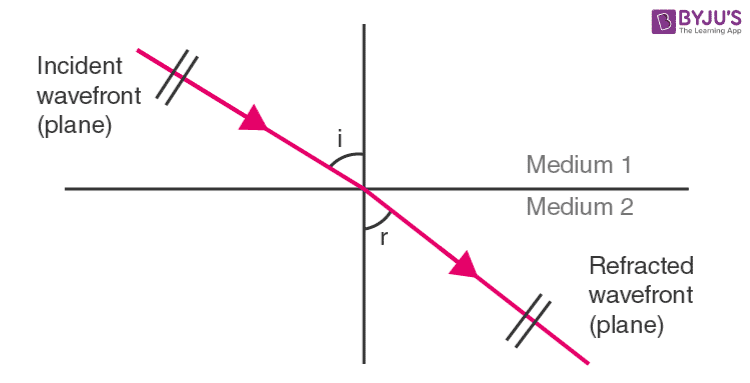• If light falls on curved surfaces

If a plane wavefront falls on a converging (or) diverging lens, the emergent light will have a spherical wavefront.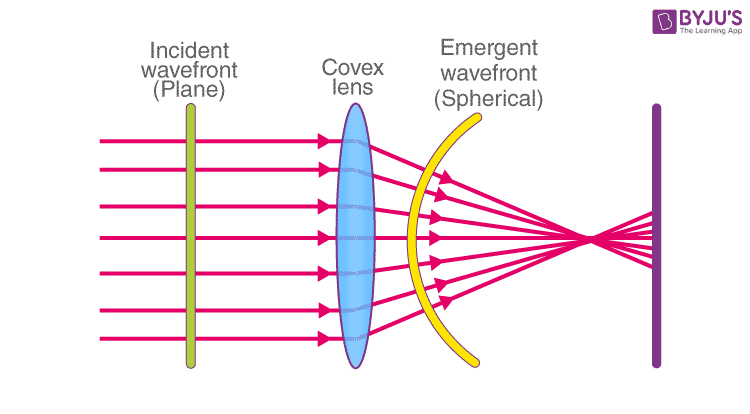A wavefront is represented by the plane

$$\begin{array}{l}y=8-\sqrt{3}x\end{array}$$
. Find the direction of the propagation of the wave.

Solution: Given,

$$\begin{array}{l}y=8-\sqrt{3}x,\end{array}$$
which is a straight-line equation. So, the wavefront can be represented as a straight line having a slope
$$\begin{array}{l}\tan \theta =-\sqrt{3}.\end{array}$$

As it has a negative slope, the wavefront is represented like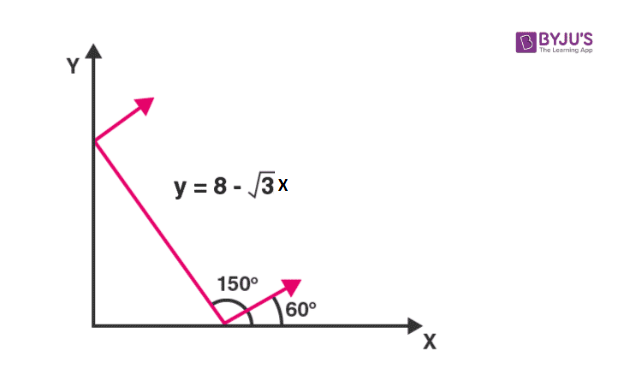∴ Wavefront makes an angle of 150° with the x-axis. As the wave must be perpendicular to the wavefront, it will make an angle of 60° with the x-axis.

### Huygen’s Principle

According to Huygen’s principle, every point on a given wavefront can be regarded as a fresh source of new disturbance and sends out its own spherical wavelets called secondary wavelets. These secondary wavelets spread out in all directions with the velocity of the wave.

A surface touching these secondary wavelets tangentially in the forward direction at any instant (Δt) gives the position and shape of the new wavefront at the instant. This is called “secondary wavefront”.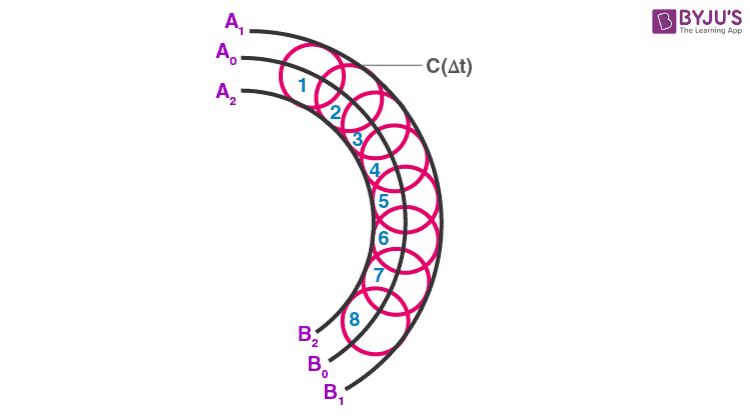As discussed in the introduction, Huygen’s principle of secondary wavelets could explain many optical phenomena like reflection, refraction, interference, and diffraction. Still, it could not explain why wavefronts of secondary wavelets are formed in the forward direction and not in the backward direction.

### Interference of Light

The effect of non-uniform energy distribution in the medium due to two superimposed light waves is called interference.

### Coherent and Incoherent Sources

Coherent Source: Two sources that emit a monochromatic light continuously with a zero (or) constant phase difference between them are called coherent sources.

Incoherent Source: The sources which do not emit light with constant phase difference are called incoherent sources.

## Video Lessons

### Young’s Double Slit Experiment### Superposition of Waves### Power Transmitted by a Wave### Ray Optics: Reflection from Plane Mirror Rapid Revision## Wave Optics – One Shot Video for JEE## Frequently Asked Questions on Wave Optics

Q1

### What is the meaning of diffraction in wave optics?

Diffraction is a phenomenon in which a wave passes through a slit or obstacle. It is known as the bending of waves around an obstacle’s end or through an aperture into the geometrical shadow of the obstacle.

Q2

### How do you differentiate between constructive and destructive interference?

Constructive interference: If two waves approach in such a way that to line up their crest together, the resultant wave will have a higher amplitude.

Destructive interference: If a crest of one wave meets the trough of another wave, the resultant wave will have a low amplitude.

Q3

### What is the meaning of wave optics in Physics?

In Physics, wave optics or physical optics is a branch of optics in which a phenomenon, where ray approximation of geometric optics is not valid such as polarization, diffraction, interference, etc.

Test your Knowledge on Wave optics